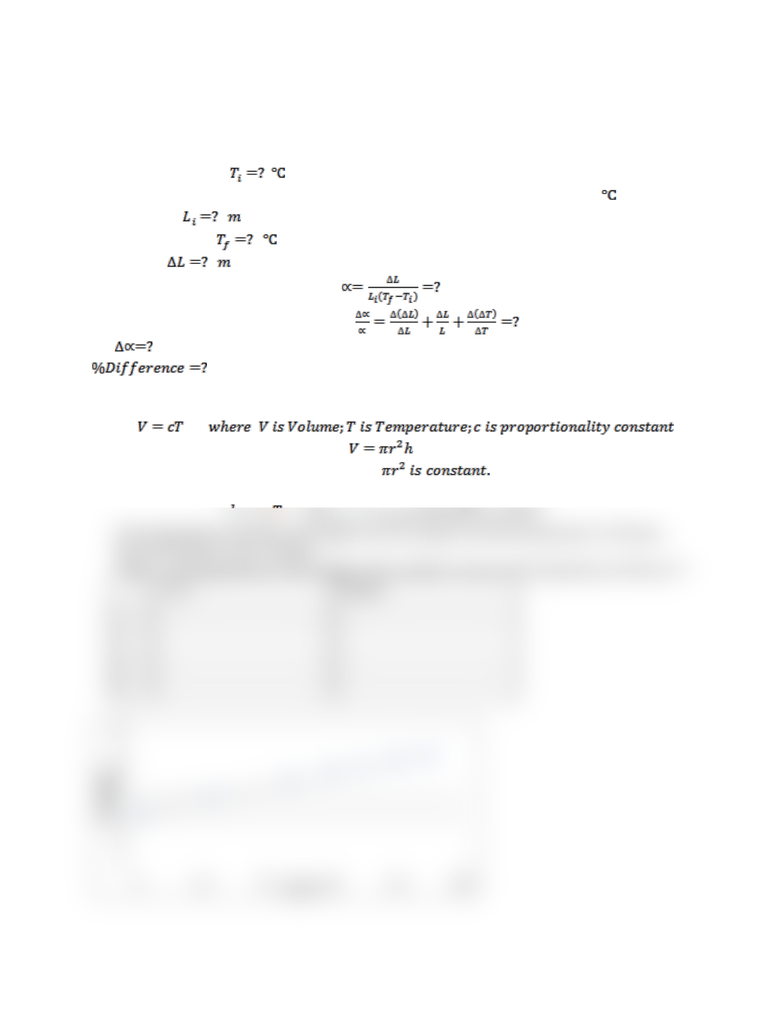Class Notes (1,200,000)
CA (650,000)
York (40,000)
PHYS (300)
Lecture

# PHYS 1010 Lecture Notes - Thermal Expansion, Multimeter

Department
Physics and Astronomy
Course Code
PHYS 1010
Professor
Marko Horbatsch

This preview shows half of the first page. to view the full 2 pages of the document.LAB 5 Thermal Physics
1. Thermal Expansion
A. Copper (for example)
calibration factor to convert it into ]
Initial Length,
Final Temperature, [similar like above]
Elongation,
Use the formula for thermal expansion,
Also use the formula to find the error,
B. For Aluminum/Steel [Do similarly]
2. Charles’ Law
We know the volume of a cylinder is, . As during this process, only height is
changing, therefore, we can conclude that
Therefore we can write the relation of Charles’ Law for this experiment as follows:
where is a proportionality constant.
If the dependence between the height
h
of the cylinder and the temperature
T
is linear,
then the Charles’ Law is verified.
Table 1: The dependence of the height of the cylinder
h
versus the temperature of the air
T
T
(oC)
h
(mm)
1. ? ?
2. ? ?
3. ? ?
4. ? ?
5. ? ?